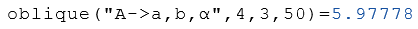# Class Oblique

Calculation of the properties of irregular triangles

## Description

The class Oblique calculates properties of irregular triangles.

## Properties

The following properties can be queried as a result or passed as an argument.

#### Description

Maße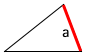a Length of $$a$$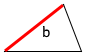b

Length of $$b$$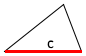c Length of $$c$$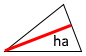ha

Height to $$a$$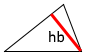hb Height to $$b$$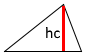hc

Height to $$c$$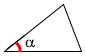alpha

$$α$$

Angle alpha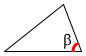beta

$$β$$

Angle beta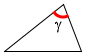gamma

$$γ$$

Angle gamma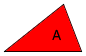A

Area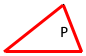P Perimeter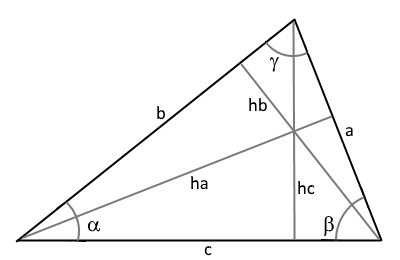## Syntax

Object = Oblique ("arg names", arguments)

Value = Oblique ("get -> arg names", arguments)

## Example

Calculation of all properties from the side lengths $$a$$ and $$b$$ and from the angle $$α$$.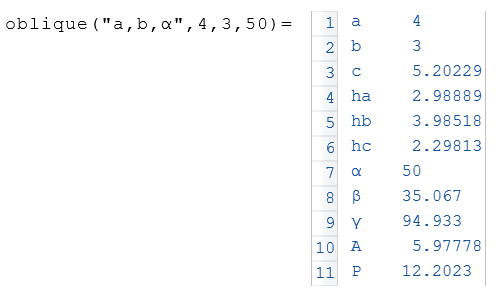The following example displays the area $$A$$ of the object $$a$$.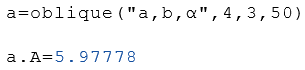In the following example, the area $$A$$ is calculated from the side lengths $$a$$ and $$b$$ and the angle $$α$$.